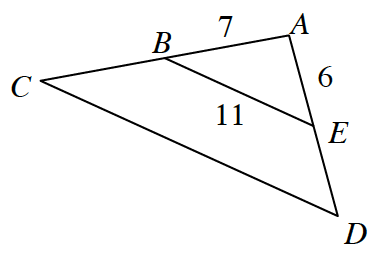### Home > CCG > Chapter Ch8 > Lesson 8.3.3 > Problem8-125

8-125.

$\overline {BE}$ is the midsegment of $\Delta ACD$, shown below.

1. Find the perimeter of $\Delta ACD$.

The perimeter of $\Delta ACD = (2)(\text{ the perimeter of } \Delta ABE)$.
Since BE is a midsegment, the two triangle have a zoom factor of $2$, that is why it is multiplied by $2$.

2. If the area of $\Delta ABE$ is $19 \text { cm}^2$, what is the area of $\Delta ACD$?

The area of $\Delta ACD = (\text{the area of } \Delta ABE)(\text { the zoom factor)}^²$.